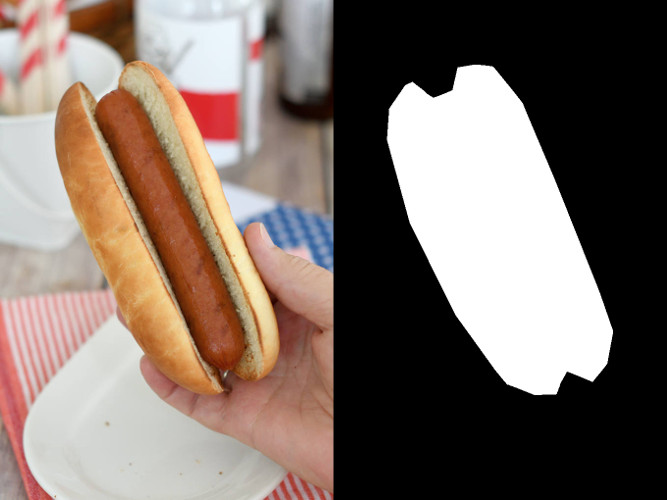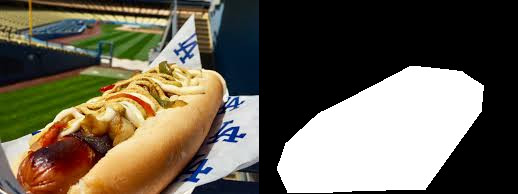Get StartedOur Voice
Our Team
Our Work
How to train Tensorflow models in Python with large augmented datasets using generatorsJohn Van Dyke
john.vd@aptusai.com
Published on Wed Feb 17 2021

Tensorflow and Keras represent a triumph in the field of machine learning. They make it possible to create novel neural network architectures to solve problems that do not have pre-built and pre-trained networks. This in addition to the flexibility of keras `.fit_generator()` has hisorically led to an easy way to use arbitrary data as both inputs and labels. In recent times, the `fit_generator` method has been deprecated, and one of the suggestions is to use tensorflow datasets.

Tensorflow datasets have a lot of upsides. They're faster, more integrated with the training loop, and can simplify much of the process of creating a model. They do however have some downsides, such as lack of flexibility. The biggest barrier to implementation, as far as I've seen, is that it's just different from the old workflow. I've relied on using generators to feed data to models because they allowed me to:

• Use datasets that won't fit in memory
• Completely control augmentation and use any generic logic on both inputs and outputs

Most of the guides I saw before beginning migration focused on very specific pieces or on very artificial/trivial datasets. Not very helpful. This article will focus on only the things needed to create a tf.data.Dataset that reads in data, and has an augmentation function that can modify both inputs and targets.

## Setup

I'm going frame this guide on the problem of image segmentation because it has large datasets and requires augmentation that modifies both the inputs and targets. Image datasets I've worked with - including document data extraction for Stax.ai and radiological imaging for Multus are HUGE. Traditional methods would've required me to have Terabytes of RAM.

The techniques shown here will work for tasks aside from image segmentation too - you may just require different augmentation techniques, or run some algorithm on the input to compute the target. The specific task I'm doing here is binary segmentation of hot dogs, i.e. pixelwise classification of hot dog vs not a hot dog - basically, to find hot dogs in images.

We'll assume our data lives in the following structure:

``````data/
data/images/
data/images/1.jpg
data/images/2.jpg
...
data/labels
data/labels/1.png
data/labels/2.png
...``````

And for simplicity, we'll assume that the problem is segmentation with only one class, so our output is just a binary mask. We'll say that the saved image files for the binary mask are 0 for the background, and 255 for the foreground to make it easier to visualize results with standard image viewing software.

Here are two examples of training images and the corresponding masks as labels:## Creating the initial Datasets

Our goal here is simply to create a list of images and labels.

``````import os
import tensorflow as tf

base_names = os.listdir('data/images')

im_names = [os.path.join('data/images', name) for name in base_names]
label_names = [os.path.join('data/labels', name.replace('jpg', 'png')) for name in base_names]

name_dataset = tf.data.Dataset.from_tensor_slices((im_names, label_names))``````

To see what we get from our dataset, we can simply do the following:

``````name_iterator = name_dataset.as_numpy_iterator()
name_batch = next(name_iterator)
print(name_batch)
=>('data/images/1.jpg', 'data/images/2.jpg')``````

Reading data is very simple, but we'll have to introduce a new concept: the `map` property of datasets. This allows us to modify the dataset and change the output. This allows us to create complex and robust pipelines to feed our models data.

``````def read_image(input_name, label_name):
input_image = tf.io.decode_jpeg(input_file)
label_image = tf.image.decode_png(label_file)
return input_image, label_image``````

If we create an iterator from `image_dataset`, it will yield tuples of images, in order `(input_image, target_image)`.

## Standardizing data

First of all, we need to have a standardized image shape, so the network will always run with the same input and output shapes.

`image_size = (256, 256)`

Now we need to do a couple of things

• Resize the inputs and targets to the common size
• Do any image pre-processing on the inputs that we want
• Change the dynamic range of the label from [0, 255] to [0, 1]
``````def standardize_dataset(image, target):
image = tf.cast(image, tf.keras.backend.floatx())
target = tf.cast(target, tf.keras.backend.floatx())
image = image - tf.math.reduce_min(image)
image = image / tf.math.reduce_std(image)
image = image - tf.math.reduce_mean(image)
# The above could be replaced with tf.image.per_image_standardization

target = target / 255.0
return image, target

standardized_dataset = image_dataset.map(standardize_dataset)``````

## Augmentation

Now we want to run a couple of basic augmentation methods, some of which will be applied to both the input and label, and some only to the label.

• Warping augmentation: applied to both
• Image degradation: applied only to image

The function that I'm using to do the affine warping allows for non-affine transforms. It's functionality is given by the tensorflow documentation as:

``````Given transform [a0, a1, a2, b0, b1, b2, c0, c1]:
(x', y') = ((a0 x + a1 y + a2) / k, (b0 x + b1 y + b2) / k), where k = c0 x + c1 y + 1``````

So to get an affine operation, all we have to do is force `c0` and `c1` to be 0. This is accomplished by masking out the last two indices. We could now construct an affine operator from the various affine operations, or we could simply add random noise to an identity operator, and tune that until we have a reasonable amount of augmentation occurring. I took the latter approach below.

Let's see how this works out in practice:

``````def augment(image, label):
image = tf.image.random_brightness(image, 0.5)
image = tf.image.random_contrast(image, 0.5, 2)
image = tf.image.random_jpeg_quality(image, 8, 60)
image = image + tf.random.normal(image_size + (3, ), 0, 0.05)

# Warping both the image and label together
initial_warp = tf.constant([1, 0, 0, 0, 1, 0, 0, 0], dtype=tf.keras.backend.floatx())
augmentation_parameters = tf.random.normal(initial_warp.shape, 0, 0.1)
affine_mask = tf.constant([0, 0, 0, 0, 0, 0, 1, 1], dtype=tf.keras.backend.floatx())
final_warp = initial_warp + augmentation_parameters

image = tfa.image.transform(image, final_warp, fill_mode='nearest', interpolation='bilinear')
label = tfa.image.transform(label, final_warp, fill_mode='nearest', interpolation='nearest')

return image, label

augmented_dataset = standardized_dataset.map(augment)``````

All parameters given above are arbitrary, but seem to give decent results. For more information on what those parameters mean, please refer to the tensorflow documentation.

## Getting into model-fitting mode

We have a few tasks left to use our dataset in a usable format for using a keras model `fit` method. First, we need to batch up the data. Second, we need to add shuffling to the dataset (this may not be needed for validation)

``````model_dataset = augmented_dataset.batch(16)  # arbitrary batch size of 16
model_dataset = model_dataset.shuffle(128)  # We need a buffer of samples to pull a random sample from. 128 is a good length for most cases

# Now we can run the model training.
model.fit(model_dataset, epochs=32)``````

## Conclusion

There's nothing inherently difficult with Tensorflow datasets. As shown above, it's possible to get most of the functionality you want from generators with datasets. There's a just a few road bumps I've run into with them that I'd like to go over in closing.

• You have to be a bit more careful with tensor datatypes and shapes a bit more than operating on numpy arrays in a generator
• There's relatively little functionality built directly into tensorflow, compared to numpy, scikit, cv2, etc. To get some more functionality, tensorflow_addons is a useful package
• Logic seems to be more difficult. As a simple example, many datasets I've used have some grayscale images and some color images. It's trivial to check the size and convert everything to the same number of channels when you're in a raw python generator. In a tensorflow generator, the shape isn't known until its being extracted, so functionality has to be invariant to the number of channels.

## Need Help?

Aptus Engineering, Inc. is an AI think tank/venutre studio and software development company that creates revolutionary novel software products using machine learning and artificial intelligence.

If you're working on a cool AI/ML project and need any help, let us know! We'd love to talk about it and see how we can help you!

You can reach our Account Manager, Lindsay at lindsay.s@aptusai.com.